# Quaternion Quantum Mechanics

## Notation Summary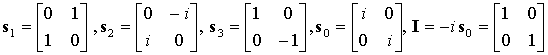(1)

Instead of  the basis s0,s1,s2,s3  use I,s1,s2,s3 .  Define: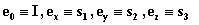(2

Also define the surface bivector units:(3)

It is possible to avoid the imaginary unit i in all equations including the Dirac equation with the help of these bivector units . The imaginary unit is mathematically convenient however, and most people are used to seeing it.  For that reason, what I’ve done is to group i with the appropriate line vector. When you see i ez for example, mentally replace it with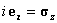Also see the section on Clifford Algebra.

Let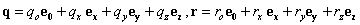be quaternions (the coefficients may be complex) then define the vector conjugate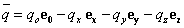(4)

and Hermitian conjugate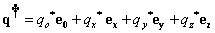(5)

In matrix form we can write: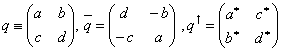(6)

### Scalar Products

There is a four-vector dot product and a space-time scalar product:(7)

### Resolve a Quaternion into Components

Given q=q0I+qxex+qyey+qzez to find q0,qx,qy,qz use:(8)

### Quaternion Exponential and Related Functions

Magnitude of a quaternion, scalar part ,vector part of quaternion ,‘unitizing’ operator U ,and angle of a quaternion.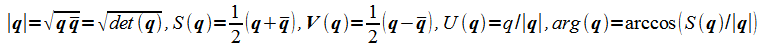(9)

Note that the magnitude of a quaternion is not necessarily real or positive. The unitizing operator U converts all quaternion to a quaternion with magnitude equal to positive one.(10)

If the quaternion is written as a 2x2 matrix then the following equivalent form is computationally useful {error: use V(q)}(11)

Also useful are the logarithm and the square root: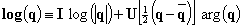(12)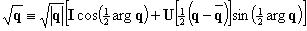(13)

### Quaternions and Space-Time Geometry

A location r in space-time is represented by a quaternion with real coefficients:(14)

r is Hermitian  r=r. (because the coefficients x,y,z,t are real)   r is said to be time-like, light-like or space-like depending on whether |r|2=det(r) is >0 ,=0 or <0.

Consider a Quaternion L of unit norm:, Then a Lorentz transform of an Hermitian quaternion r is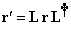(15)

Eq (15) represents only homogenous Lorentz transforms that are proper(preserves spatial parity or ‘handness’), and orthochronous(preserves time direction)). It’s obvious that multiplying L by a complex number of the form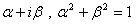won’t change the Lorentz transform at all.  It is most convenient to choose the parameters so that |L|=1 and the scalar part of L is pure real.  Or det(L)=+1 and Im(Trace(L))=0.

If L happens to be Hermitian: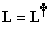then L effects a pure velocity transform If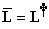then we have a pure 3D rotation (see below)  .

The Lorentz transform preserves the space-time norm of any quaternion: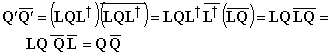(16)

### 3d rotation

To rotate thru the angle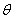about a unit axis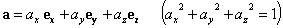(note that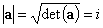, alternatively one could use the axis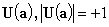that would remove the factor of  i in the rotation formula and introduce a factor of i in the Lorentz formula but that would spoil the geometric interpretation. Note that

i a is the unit bivector defining the plane of rotation)

use the quaternion: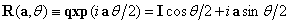Then the rotation is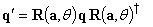(Right hand rule: point thumb along a curl fingers in direction of positive)

### Lorentz Transform

To effect a Lorentz transform of speed v along the unit axis:(That is: transform to a frame where an object that was at rest, in the initial reference frame now appears to move at velocity v in the new reference frame)

First, let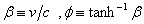, then use the quaternionThe Lorentz transform of q is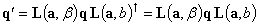For the space-time event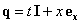, the coordinates will transform to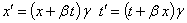(For a velocity in the opposite direction (-v/c) replace a with –a in the expression for L)

Find the Lorentz transform that ‘rotates’ space-time event A into B. That is for unit Hermitian quaternions A and B find L such that: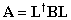Then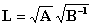(Note that A and B must be Hermitian and time-like)

### Canonical form  Lorentz Transform

As mentioned det(L)= +1 and the scalar part of L is pure real.  With these restrictions, the most general form of L is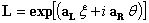(17)

where aL and aR are real  (Hermitian) unit three-vector quaternions  of the formand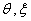are real numbers. These six independent parameters together with det(L)=+1 and   Im (Tr(L))=0 determine the eight components of L, Use the quaternion logarithm function (12) to find these parameters. The usual rule for the product of exponentials generally does not work for quaternion exponentiation, however through Singular Value Decomposition L can be factored into: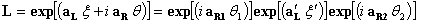(18)

That is  any Lorentz transform can be resolved into a rotation followed by a boost along the z axis followed by another rotation. Formulas for the parameters in (18) are exceedingly complicated and won’t be written here. They can be obtained from any good symbolic algebra program—just apply SVD to a general complex 2x2 matrix.

So to get a product of Lorentz quaternions L1 L2..Ln into the form (17), use the logarithm function. To get the canonical form

(18) use  singular value decomposition.(19)

Let S(t,x,y,z) be a scalar valued function of the coordinates that is, some field takes on the value S(t,x,y,z) at the space time point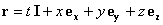. The gradient operator connects the values at neighboring points. Let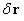be the usual small displacement from r then in terms of the ordinary gradient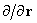we write:(20)

To get the same expression in terms of the quaternion gradients of (19) we use the scalar dot product  (7a)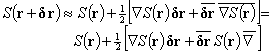(21)

(The second line is a notation convention)

Next, consider an Hermitian quaternion-valued field Q(r):(22)

Let a be the three vector  with real components (ax,ay,az),The quaternion gradient combines the gradient, divergence, curl :(23)

For a anti-hermitian quaternion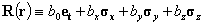(24)(25)

The quaternion gradient can be defined as a volume integral: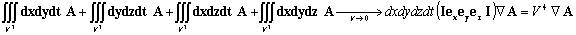(26)

Here, dx,dy,dz,dt are quaternions  understood to be dx ex with dx a scalar and so forth.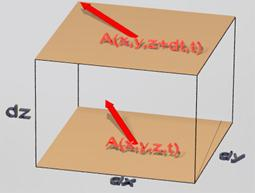## Quaternion Dirac Equation

Reference:  J Scott: Complex four-vectors and the Dirac equation

A good paper. Some of his terminology is a little different from mine.  For example his term quaternion corresponds to Hermitian quaternion.

In the original form as described in the Principles of Quantum Mechanics, Dirac defined the following 4x4 matrices, Portray them here in block-partitioned format.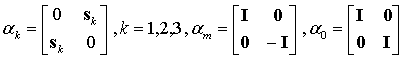(27)

The four-vector wave functionevolves according to: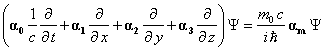(28)

We can also write this as:(29)

### Solutions

Let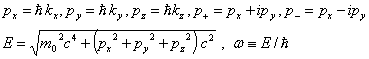(30)

Define: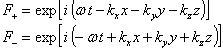(31)

Then(32)

### Quaternion form

Define the following quaternion: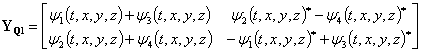(33)

Then either (28) or (29) is equivalent to: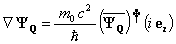(34)

### Direct Form

To solve (34), apply the Lorentz transform to the particle at rest solution: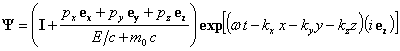(35)

(E,p,w are defined in(30))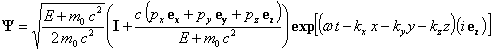(This normalization makes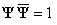).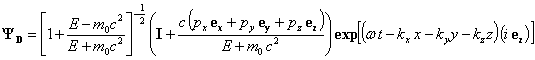(This normalization makes the probability density r=1.  see below).

There is also a time reversed negative energy solution to (34).(36)

Also note that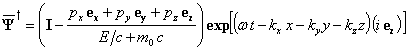(37)

Through simple algebra, we get the following variations on (34).(38)

Furthermore, let(39)

Eq (34), (38b) and definitions (39) can be combined into:(40)

The advantage of the last form is that it avoids the conjugate of the wave function. It doubles the number of equations and might be explored as a generalization of the Dirac equation.  Eq (39) and 35 (35) are special solutions. These equations were first discovered by Lanczos and Proca in the 1930’s, later rediscovered by others.

Another advantage of (40) is that we can most easily relate it to the QRW process.

### Probability Current

Let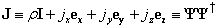(41)

be the probability current. J is an Hermitian quaternion so the components r, j are real.  (note that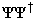is Hermitian but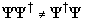).

We should be able to prove from the Dirac equation (34) that: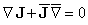(42)

but I can't find a direct proof. The best I could do was to numerically differentiate randomly chosen quaternions and check (42) that way.
Or, argue that (28) and (34) are equivalent, so they should both lead to (43) below. And thanks to Dirac, we know that (28) leads to (43).

From the four-vector dot product formula (7a) and (42)it follows that: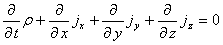(43)

To summarize: the probability density is the scalar part of the quaternion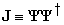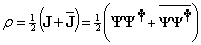(44)

.

The probability current  is the vector part of J:(45)

(Second equality: conjugate(Q)=-Q if Q has no scalar part)